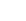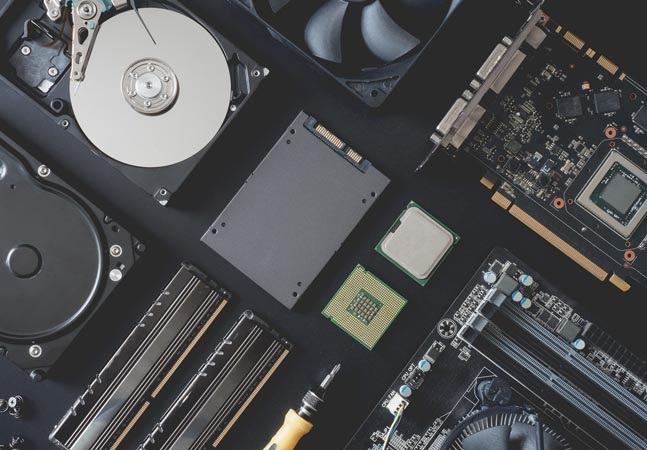Posey's Tips & Tricks

### Machine Learning 101: The Building Blocks To Get Started

It may be the buzziest tech trend of the moment, but machine learning is no easy matter. Before you jump into writing machine learning algorithms, here are the basics you need to start a project.One of the hottest tech trends right now is machine learning. It seems that artificial intelligence (AI) and machine learning algorithms are being embedded into nearly every kind of software application imaginable.

Even so, I have found that the inner workings of machine learning are a bit fuzzy to many IT pros. In fact, I have recently had several people ask me questions pertaining to how machine learning works, or how they can build their own machine learning apps.

In all honesty, I would have to write a fairly long book in order to do justice to the topic of machine learning. But while a single column might not give me the opportunity to go into as much technical depth as I would like, I want to give you an overview of how machine learning works. As I do, keep in mind that I am generalizing and that there are many different types of machine learning algorithms.

A simple machine learning algorithm starts out with a predictive mathematical model. This is really just a mathematical function. In math, a function takes input values and then calculates output values based on that input. In the case of machine learning, the function should be based on what you expect to happen. Let me give you an example.

Let's pretend for a moment that we want to use machine learning to predict a certain behavior. This behavior could be anything; the specifics are irrelevant to the example. In the interest of keeping things simple, let's suppose that my best guess is that the end result will be a doubling of my input value. I might express this as:

`F(x) = 2X`

In other words, if X is 2, then F(x) would equal 4. If X is 3 then F(x) would be six. Because the function is linear, I could express it in slope-intercept form (Y=MX+B), but let's keep things simple.

At this point, I have an extremely simple predictive model that says that whatever my input value is, the output will be double. Remember, though, that this model was based on my best guess for how I think things will play out. The model may or may not be right.

The next step in the process would be to gather some real-world data and see how my mathematical model stacks up. Let's suppose for a moment that we observed the following real-world input and output values:

```Input	Output
2	5
3	7.5
4	10```

In the real world, we would of course need to observe a lot more data, but these three value sets will work for the sake of example. In this case, we can see that our predictive model was incorrect. The function says that an input value of 2 should yield an output value of 4, but we are getting an output value of 5 instead. Similarly, the function says that an input value of 3 should yield an output value of 6, but we are getting 7.5.

In the old days, this type of situation would have meant that we needed to come up with a more accurate function. In the world of machine learning, however, the algorithm takes the real-world data that has been observed, and then works backward to create a mathematical function that can explain the data. In this case, that function would be:

`F(x) = 2.5X`

So my initial model was somewhat close to being on track, but by observing real-world data, we discovered that the model needed to be revised. That's exactly what machine learning does.

Now, here is where things get interesting. Let's pretend that we collected some additional real-world data and found it to contain the following input/output values:

```Input	Output
2	5
3	7.5
4	10
5	192
6	15
5	12.5
7	17.5```

The lesson here is that real-world observations cannot always be neatly mathematically modeled. There are a couple of different problems with the data here. First, notice that the input value of 5 is listed twice. That isn't a problem in and of itself, but what is a problem is that there are two different results. A mathematical function cannot give two different answers for the same input.

The other problem is that the first occurrence of 5 as an input value yields an output of 192. This is way outside the range of what would be expected based on both the model and the data. Clearly, this is either bad data or an extreme outlier.

These and similar problems can be common when you (or an algorithm) are trying to model real-world data. Therefore, most of the machine learning algorithms that I have seen use statistical analytics for the purpose of filtering out data that is way outside of the norm.

As previously noted, the basis of this type of machine learning algorithm is that it modifies a mathematical function as more and more data becomes available. The modification process happens more than once. Each time more data is collected, that data is analyzed and the function is refined to reflect the new data. (Keep in mind that I am only discussing machine learning in the most basic sense. Modern machine learning techniques can be far more complex.)

If you want to try your hand at building a machine learning algorithm, you will, of course, need to know how to write code. Python seems to be a popular choice when it comes to do-it-yourself machine learning. You will also need to have a strong background in math, specifically with regard to matrices, differential equations, statistics and linear algebra.

Brien Posey is a 21-time Microsoft MVP with decades of IT experience. As a freelance writer, Posey has written thousands of articles and contributed to several dozen books on a wide variety of IT topics. Prior to going freelance, Posey was a CIO for a national chain of hospitals and health care facilities. He has also served as a network administrator for some of the country's largest insurance companies and for the Department of Defense at Fort Knox. In addition to his continued work in IT, Posey has spent the last several years actively training as a commercial scientist-astronaut candidate in preparation to fly on a mission to study polar mesospheric clouds from space. You can follow his spaceflight training on his Web site.